• Turtle是Python的一种功能，如绘图板，它使我们可以命令Turtle在其上进行绘制！可以使用诸如turtle.forward(…)和turtle.right(…)之类的函数来移TurtleTurtle是一种初学者友好的类库，可通过运行一些基本命令并...


Turtle是Python的一种功能，如绘图板，它使我们可以命令Turtle在其上进行绘制！可以使用诸如turtle.forward(…)和turtle.right(…)之类的函数来移Turtle。Turtle是一种初学者友好的类库，可通过运行一些基本命令并以图形方式查看Turtle来学习Python。就像绘图板一样，您可以在其上绘制。turtle模块可以以面向对象和面向过程的方式使用。为了进行绘制，Python turtle提供了许多功能和方法，即前进，后退等。一些常用的方法是：forward(x)：将笔沿x方向向前移动。 向后(x)：将笔向后移动x单位。 right(x)：沿顺时针方向将笔旋转角度x。 left(x)：沿逆时针方向将笔旋转角度x。 penup()：停止绘制龟笔。 pendown()：开始绘制龟笔。 在本文中，将编写代码，借助Turtle编程绘制一朵花。如下图所示。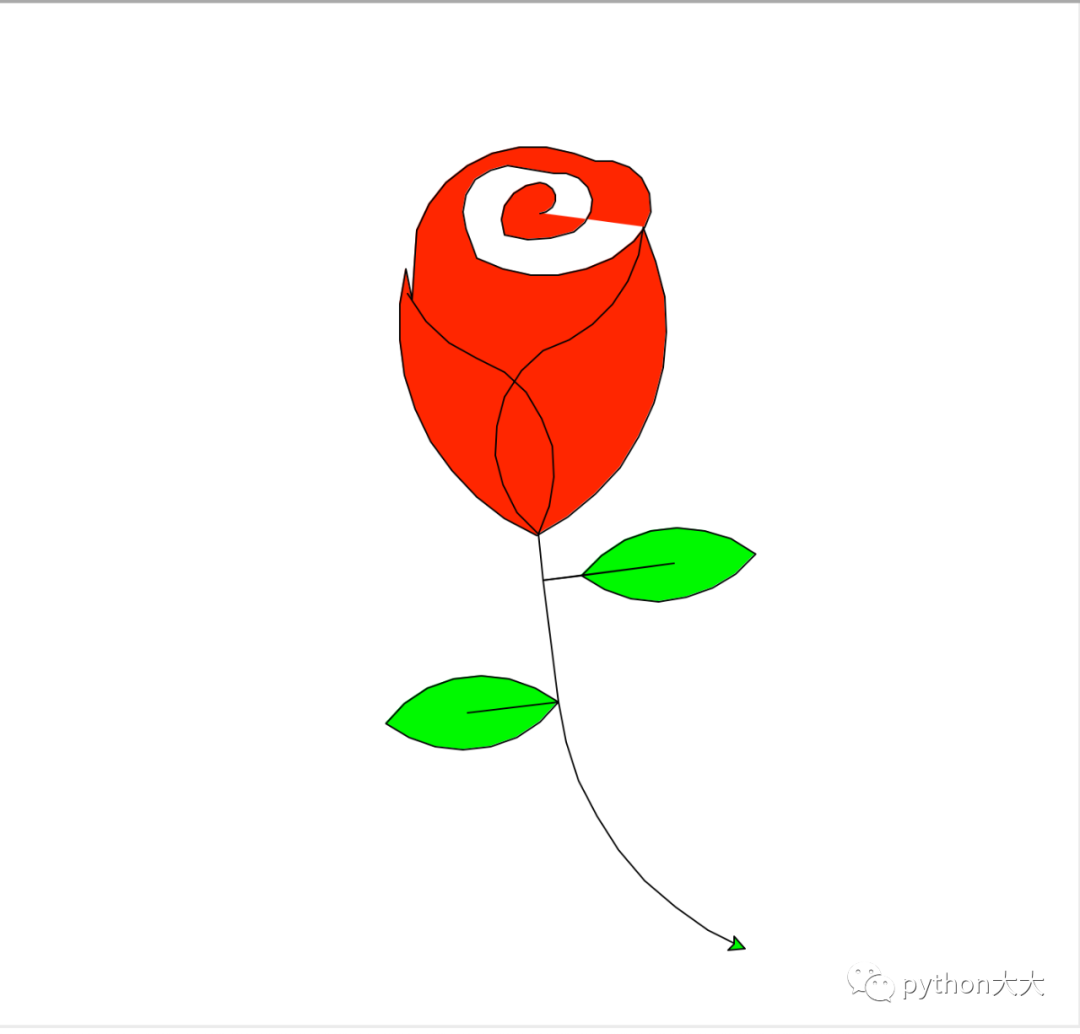方法：导入Turtle模块设定Turtle速度使用循环避免不必要的代码重复。用特定的坐标绘制每个步骤下面是具体代码：import turtle # Set initial positionturtle.penup ()turtle.left (90)turtle.fd (200)turtle.pendown ()turtle.right (90) # flower baseturtle.fillcolor ("red")turtle.begin_fill ()turtle.circle (10,180)turtle.circle (25,110)turtle.left (50)turtle.circle (60,45)turtle.circle (20,170)turtle.right (24)turtle.fd (30)turtle.left (10)turtle.circle (30,110)turtle.fd (20)turtle.left (40)turtle.circle (90,70)turtle.circle (30,150)turtle.right (30)turtle.fd (15)turtle.circle (80,90)turtle.left (15)turtle.fd (45)turtle.right (165)turtle.fd (20)turtle.left (155)turtle.circle (150,80)turtle.left (50)turtle.circle (150,90)turtle.end_fill () # Petal 1turtle.left (150)turtle.circle (-90,70)turtle.left (20)turtle.circle (75,105)turtle.setheading (60)turtle.circle (80,98)turtle.circle (-90,40) # Petal 2turtle.left (180)turtle.circle (90,40)turtle.circle (-80,98)turtle.setheading (-83) # Leaves 1turtle.fd (30)turtle.left (90)turtle.fd (25)turtle.left (45)turtle.fillcolor ("green")turtle.begin_fill ()turtle.circle (-80,90)turtle.right (90)turtle.circle (-80,90)turtle.end_fill ()turtle.right (135)turtle.fd (60)turtle.left (180)turtle.fd (85)turtle.left (90)turtle.fd (80) # Leaves 2turtle.right (90)turtle.right (45)turtle.fillcolor ("green")turtle.begin_fill ()turtle.circle (80,90)turtle.left (90)turtle.circle (80,90)turtle.end_fill ()turtle.left (135)turtle.fd (60)turtle.left (180)turtle.fd (60)turtle.right (90)turtle.circle (200,60)turtle.done()完整代码在关注本公众号后,发送“画玫瑰"免费获取。并有免费代码讲解赠送。


展开全文• 提到程序员，可能每个同学都认识一个——爱穿格子衬衫、冲锋衣，爱宅着敲代码的程序员或者准程序员，他们仿佛永远在bug和debug的路上，还总爱自黑自己是“单身狗”，你可能认为他们是这样的：这样的还有这样的其实...提到程序员，可能每个同学都认识一个——爱穿格子衬衫、冲锋衣，爱宅着敲代码的程序员或者准程序员，他们仿佛永远在bug和debug的路上，还总爱自黑自己是“单身狗”，你可能认为他们是这样的：这样的还有这样的其实不然一些程序员表面上看似孤身一人其实人家已经悄咪咪的找了女朋友单身的朋友依旧独自行走在冬夜的冷风中总不能用代码写一个女朋友出来吧程序员版本《恋爱宝典》你值得拥有表白的错误示范大胆冒失型：缺根筋型：用力过猛型：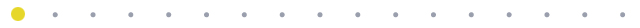其实程序员们也有属于自己的浪漫啦，譬如Python、C、Java、C#等等等~都有很强悍的撩妹功能。本期，小编为大家带来使用Python表白的各种姿势。如果再学不会，那就真的是凭自己的本事单身了！01初阶版：一行代码画出爱心♡使用语言：Python代码：print('\n'.join([''.join([('Love umd'[(x-y)%8]if((x*0.05)**2+(y*0.1)**2-1)**3-(x*0.05)**2*(y*0.1)**3<=0 else' ')for x in range(-30,30)])for y in range(15,-15,-1)])效果图：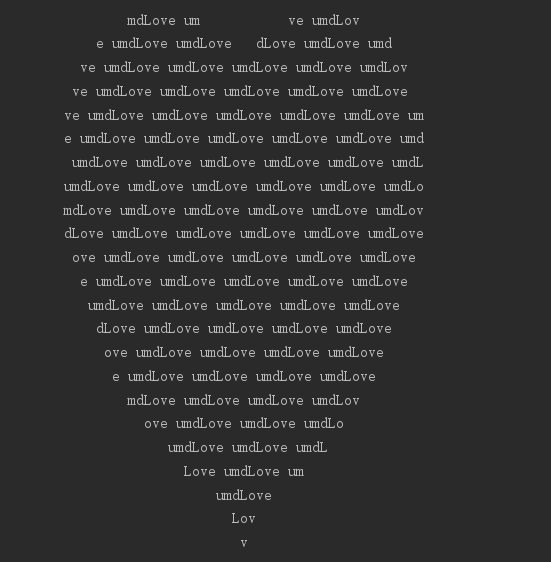02进阶版：python + 数学= LOVE YOU使用语言：Python代码： import matplotlib.pyplot as pltimport seabornimport numpyl = numpy.arange(0, 4, 0.01)L = 1.0 / ltheta = numpy.arange(-4, 4, 0.01)o = 3.0 * numpy.cos(theta)O = 3.0 * numpy.sin(theta)v = numpy.arange(-4, 4, 0.01)V = numpy.abs(-2.0 * v)e = numpy.arange(-3, 3, 0.01)E = -1.0 * numpy.abs(numpy.sin(e))y = numpy.arange(-10, 10, 0.01)Y = numpy.log2(numpy.abs(y))u = numpy.arange(-4, 4, 0.01)U = 2.0 * u ** 2points = []for heartY in numpy.linspace(-100, 100, 500):    for heartX in numpy.linspace(-100, 100, 500):        if ((heartX*0.03)**2+(heartY*0.03)**2-1)**3-(heartX*0.03)**2*(heartY*0.03)**3 <= 0:            points.append({"x": heartX, "y": heartY})heart_x = list(map(lambda point: point["x"], points))heart_y = list(map(lambda point: point["y"], points))fig = plt.figure(figsize=(13, 7))ax_L = fig.add_subplot(2, 4, 1)ax_O = fig.add_subplot(2, 4, 2)ax_V = fig.add_subplot(2, 4, 3)ax_E = fig.add_subplot(2, 4, 4)ax_Y = fig.add_subplot(2, 4, 5)ax_O_2 = fig.add_subplot(2, 4, 6)ax_U = fig.add_subplot(2, 4, 7)ax_heart = fig.add_subplot(2, 4, 8)plt.plot(colos='tomato')ax_L.plot(l, L)ax_O.plot(o, O)ax_V.plot(v, V)ax_E.plot(E, e)ax_Y.plot(y, Y)ax_Y.axis([-10.0, 10.0, -10.0, 5.0])ax_O_2.plot(o, O)ax_U.plot(u, U)ax_heart.scatter(heart_x, heart_y, s=10, alpha=0.5)seaborn.set_style('whitegrid')plt.show()效果图：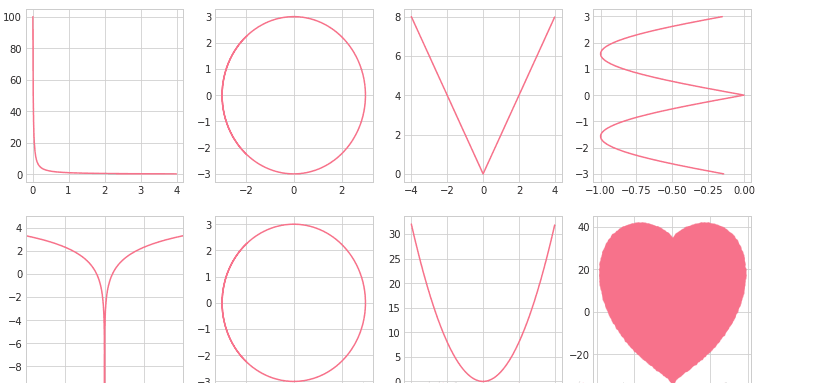03终极版：用Python画一只玫瑰使用语言：Python代码：import turtleimport time# writing txtturtle.hideturtle()turtle.penup()turtle.goto(130, 50)# turtle.pendown()turtle.color("blue")turtle.write("亲爱的，给你画个东西", font=("Times", 18, "bold"))time.sleep(2)turtle.goto(180, 10)turtle.write("开始了哈~", font=("Times", 18, "bold"))time.sleep(2)turtle.goto(200, -20)turtle.write("Ready?", font=("Times", 18, "bold"))time.sleep(2)turtle.goto(215, -50)turtle.write("go!", font=("Times", 18, "bold"))time.sleep(2)# turtle.end_fill()## 设置初始位置turtle.goto(0, 0)turtle.color("black")turtle.penup()turtle.left(90)turtle.fd(200)turtle.pendown()turtle.right(90)# 花蕊turtle.fillcolor("red")turtle.begin_fill()turtle.circle(10, 180)turtle.circle(25, 110)turtle.left(50)turtle.circle(60, 45)turtle.circle(20, 170)turtle.right(24)turtle.fd(30)turtle.left(10)turtle.circle(30, 110)turtle.fd(20)turtle.left(40)turtle.circle(90, 70)turtle.circle(30, 150)turtle.right(30)turtle.fd(15)turtle.circle(80, 90)turtle.left(15)turtle.fd(45)turtle.right(165)turtle.fd(20)turtle.left(155)turtle.circle(150, 80)turtle.left(50)turtle.circle(150, 90)turtle.end_fill()# 花瓣1turtle.left(150)turtle.circle(-90, 70)turtle.left(20)turtle.circle(75, 105)turtle.setheading(60)turtle.circle(80, 98)turtle.circle(-90, 40)# 花瓣2turtle.left(180)turtle.circle(90, 40)turtle.circle(-80, 98)turtle.setheading(-83)# 叶子1turtle.fd(30)turtle.left(90)turtle.fd(25)turtle.left(45)turtle.fillcolor("green")turtle.begin_fill()turtle.circle(-80, 90)turtle.right(90)turtle.circle(-80, 90)turtle.end_fill()turtle.right(135)turtle.fd(60)turtle.left(180)turtle.fd(85)turtle.left(90)turtle.fd(80)# 叶子2turtle.right(90)turtle.right(45)turtle.fillcolor("green")turtle.begin_fill()turtle.circle(80, 90)turtle.left(90)turtle.circle(80, 90)turtle.end_fill()turtle.left(135)turtle.fd(60)turtle.left(180)turtle.fd(60)turtle.right(90)turtle.circle(200, 60)time.sleep(2)turtle.penup()turtle.color("blue")turtle.goto(180, -100)turtle.write("umd~送给你~", font=("Times", 18, "bold"))time.sleep(20)效果图：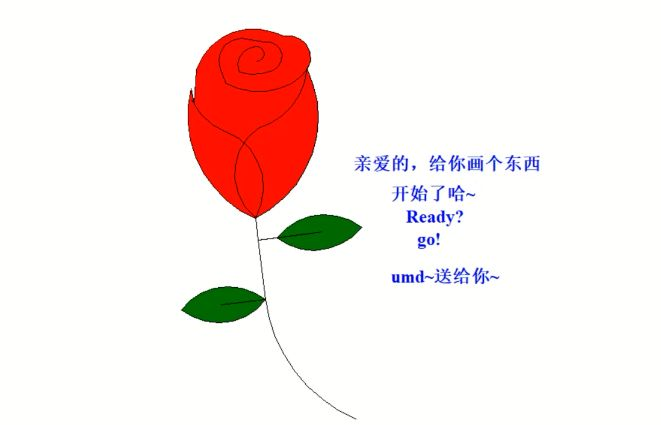怎么样？各位霸霸们，get到新技能了吗还有什么新奇的招数热烈欢迎程序猿霸霸们在留言板给大家提点一番重庆大学UMD研究生网络文化工作室编辑丨赵奉超图源 | 梦游家、网络审核丨江   欣指导丨陈大勇、裴光术

展开全文• 本篇进入Python第二章的学习，下面是主要内容深入理解Python语言实例2：Python蟒蛇绘制模块1：turtle库的使用turtle程序语法元素分析深入理解Python语言计算机技术的演进1946-1981 计算机系统结构时代解决计算能力...
本篇进入Python第二章的学习，下面是主要内容深入理解Python语言实例2：Python蟒蛇绘制模块1：turtle库的使用turtle程序语法元素分析深入理解Python语言计算机技术的演进1946-1981 计算机系统结构时代解决计算能力问题1981-2008 网络和视窗时代解决交互问题2008-2016 复杂信息系统时代解决数据问题2016- 人工智能时代解决人类的问题不同程序语言的初心和适用对象C语言学习内容： 指针、内存、数据结构语言本质： 理解计算机系统结构解决问题： 性能问题Java学习内容：对象、跨平台、运行时语言本质：理解主客体关系解决问题：跨平台C++学习内容：对象、多态、继承语言本质：理解主客体关系解决问题：大规模程序VB学习内容：对象、按钮、文本框语言本质：理解交互逻辑解决问题：桌面应用Python学习内容：编程逻辑、第三方库语言本质：理解问题的求解解决问题：各类问题编程语言种类机器语言一种二进制语言，直接适用二进制代码表达指令CPU可以直接执行，与具体CPU型号有关汇编语言一种二进制代码直接对应助记符的编程语言汇编语言与CPU有关，程序不通用高级语言更接近自然语言，同时更容易计算高级语言代码与具体CPU无关，编译后运行超级语言粘性整合已有程序，具备庞大计算生态编程是集成开发实例2：Python蟒蛇绘制#PythonDraw.py
举一反三程序参数改变python蟒蛇的颜色python蟒蛇的长度python蟒蛇的方向模块1：turtle库的使用turtle库介绍1969年诞生，主要用于程序设计入门python语言的标准库之一入门级的图形绘制函数库turtle的绘图窗体turtle的一个画布空间最小单位是像素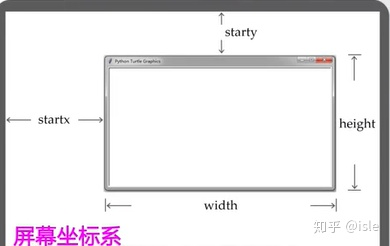turtle.setup(width,height,startx,starty)
#设置窗体大小和位置
#后四个参数后两个可选
#这个函数不是必须的turtle空间坐标体系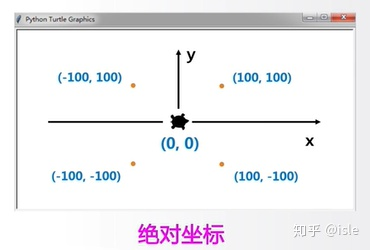turtle.goto(x,y)
#让海龟到（x,y）的位置例子import turtle
turtle.goto(100,100)
turtle.goto(100,-100)
turtle.goto(-100,-100)
turtle.goto(-100,100)
turtle.goto(0,0)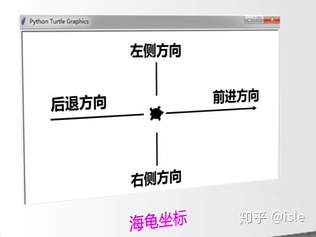turtle.bk(d)
#向海龟正后方向行走
turtle.fd(d)
#向海龟的正前方向行走
turtle.circle(r,angle)
#以海龟当前位置左侧的一个点为圆心旋转运行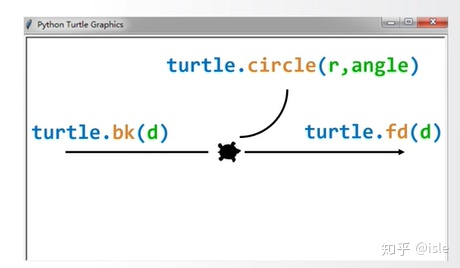turtle角度坐标体系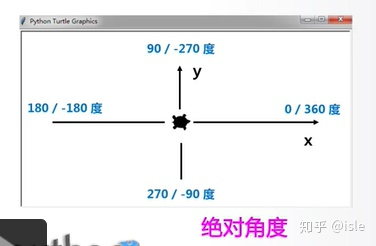turtle.seth(angle)
#改变海龟的行进方向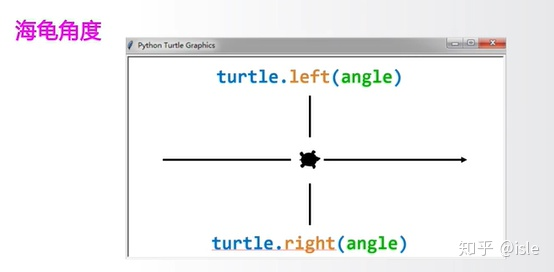turtle.left(angle)
turtle.right(angle)例子import turtle
turtle.left(45)
turtle.fd(150)
turtle.right(135)
turtle.fd(150)
turtle.left(135)
turtle.fd(150)RGB色彩体系RGB指红绿蓝三个通道的颜色组合覆盖视力所能感知的所有颜色RGB每色取值范围是0-255整数或者0-1小数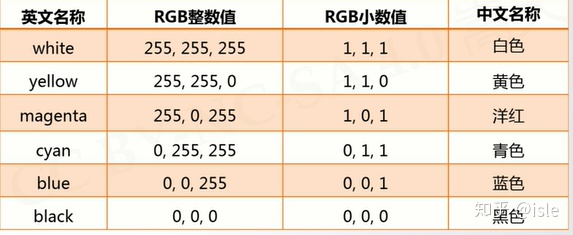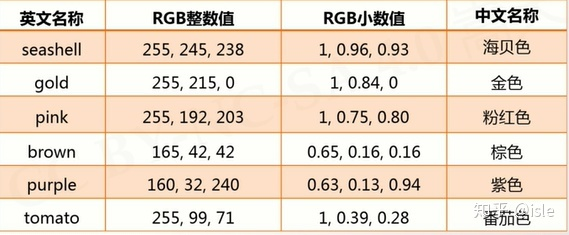turtle.colormode(mode)
#mode=1.0 RGB小数模式
#mode=255 RGB整数模式turtle程序语法元素分析库引用：扩充python程序功能的方式使用import保留字完成，采用<a>.<b>()编码风格import<库名><库名>.<函数名>(<函数参数>)import的更多用法from<库名>import<函数名>from<库名>import*<函数名>(<函数参数>)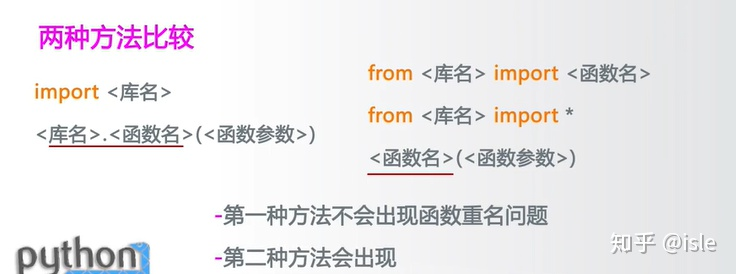import<库名> as<库别名><库别名>.<函数名>(<函数参数>)python画笔控制函数turtle.penup()/turtle.pu()turtle.pendown()/turtle.pd()上述代码成对出现turtle.pensize(width)画笔宽度turtle.pencolor(color)画笔颜色turtle.pencolor("purple)turtle(0.63,0.13,0.94)turtle((0.63,0.13,0.94))总结penup()
pendown()
pensize(width)
pencolor(colorstring)
pencolor(r,g,b)
pencolor((r,g,b))python运动控制函数turtle.forward(d)/turtle.fd(d)向前行进d:行进距离，可以为负数turtle.circle(r,extent=None)根据半径r绘制extent角度的弧形r:绘制的半径extent:绘制的角度默认情况下，圆心在左侧python方向控制函数turtle.setheading(angle)/turtle.seth(angle)改变行进方向angle:改变方向为一个绝对角度turtle.left(angle)turtle.right(angle)angle：在海龟当前行进方向上旋转的角度循环语句按照一定次数循环执行一组语句for<变量>表示每次循环的计数，0到<次数>-1range(N)产生0到N-1的整数序列，共N个range(M,N)产生M到N-1的整数学列，共N-M个
展开全文• python学习心得 Python实训已经接近尾声，在一周的实训过程中，我领略了世界上最流行的编程语言——Python...例如学会了Python基本图形绘制及turtle库的使用，这是Python学习上一个新的开始。首先，使用import turtl...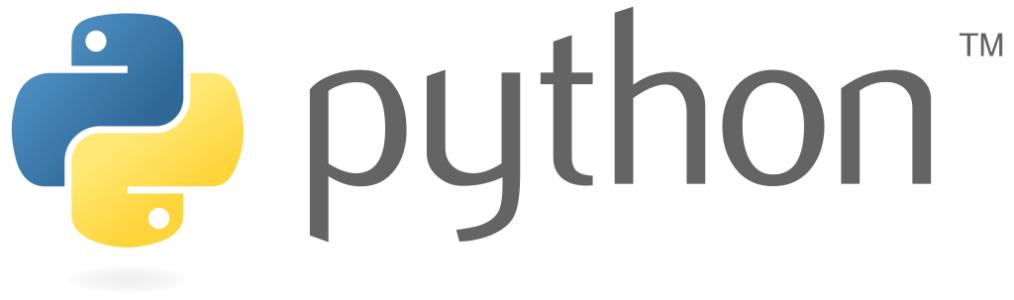python学习心得       Python实训已经接近尾声，在一周的实训过程中，我领略了世界上最流行的编程语言——Python的魅力。       在Python实训周中，不但加深了以往对于Python基础语法的认识和运用，更重要的是，在原来的基础上，学到了新的东西。例如学会了Python基本图形绘制及turtle库的使用，这是Python学习上一个新的开始。首先，使用import turtle as 语句导入turtle 库，在调用turtle库中的函数，采用turtle.函数名() 的代码风格，利用 turtle.pendown() 构建画笔， turtle.pensize(width) 设置画笔宽度， turtle.color(“red”) 设置画笔颜色，turtle.done() 设置窗口等待后关闭，由此便完成了一幅图形的绘制。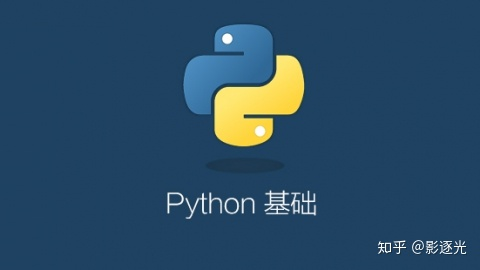这次Python实训不仅是对往期学习的巩固和加深，更是一次“温故知新”的过程，在老师的带领下，对现有知识又获得了新的认识，对于之前学过的Python的基本数据类型、程序的控制结构、函数的代码复用、正则表达式、组合数据类型、文件和数据格式化等，都有了新的认识与理解，并深刻感受到Python简洁却强大、简单却专业的强大魅力。       总而言之，本次实训让我受益匪浅，非常感谢老师的耐心指导，让我学有所得。
展开全文• 首先，我们来画脸框，代码实现如下： turtle.speed(5) turtle.setup(500, 500) turtle.pensize(5) turtle.right(90) turtle.penup turtle.fd(100) turtle.left(90) turtle.pendown turtle.begin_fill turtle....
• 乐学趣学Py●08：while循环的应用●Python趣味小百科Python程序中有一个有彩蛋，在IDLE Pythton模式下输入import ...这首小诗表明了用Python编写代码时遵循的原则，其中第四句是：Complex is better than complicate...
• 现在让我们看代码： import turtlet = turtle.Pen()turtle.bgcolor("black")colors = ["red", "blue", "green", "yellow"]for x in range(100): t.pencolor(colors[x%4]) t.forward(x) t.left(55) 4种颜色的列表...
• 具体代码展示如下： import turtlet = turtle.Pen()turtle.bgcolor("gray")turtle.speed(0)colors = ["orange", "blue", "red", "yellow" for x in range(1000): t.pencolor(colors[x%4]) t.circle(x) # ...python大作业
• turtle库是画图库库调用库调用可以有三种方法 使用时可以import turtle，类似于c语言开头的调用头文件，每调用一个函数就要加turtle，如turtle.setup();turtle.seth()……或者是from turtle import*,这样后面可以...
• 6月14日学习笔记今天李老师在群里提出了一个问题“如何实现turtle同步画坐标轴？”，大家围绕着个问题进行了讨论。一提到同步画我就想到scratch的多角色并行编程，包括vb，也都很容易实现并行。这或许是可视化编程的...伪代码的简单例子
• 太极的图案想必你很清楚吧！但是让自己画出来也不一定很...import turtleturtle.speed(10)turtle.pendown()turtle.circle(100,180)turtle.circle(200,180)turtle.circle(100,-180)turtle.fillcolor('black')turtle...
• 第6行代码turtle.bgcolor是设置海龟库画笔的背景颜色，括号内设置为蓝色。注意：表示颜色的单词要放在单引号内。对照图形化代码！一个蓝色背景，红色画笔的六边形完成了！有时候输入颜色单词比较麻烦，怎么办呢？...
• ATM接受该卡并读它上面的分行代码和卡号。 ATM要求储户输入密码；储户输入自己的密码“1234”等数字。 ATM请求总行验证卡号和密码；总行要求“39”号分行核对储户密码，然后通知ATM说这张卡有效。 ATM要求储户选择...
• 使用turtle设置颜色的命令如下：turtle.color('#FF8EFF', '#2F0000')第一个颜色为画笔颜色，第二个颜色为填充颜色，'#FF8EFF', '#2F0000'为颜色代码。为了有个直观的印象，下图中的所有颜色turtle都可以使用。简单画...
• 利用python画一棵漂亮的樱花树，turtle画图代码大全,此处感谢知乎大佬小白此处感谢知乎大佬小白练手练习一下比较流行的turtle（海龟库）画一棵漂亮的樱花树，效果如下：ps: 是动态画出的哈哈代码如下：1 import ...
• 一、从基本的正方形开始引入turtle模块并创建Pen对象：>>> importturtle>>> t = turtle.Pen()前面我们用来创建正方形的代码如下：>>> t.forward(50)>>> t.left(90)>>> t.forward(50)>>> t.left(90)...
• 【实例简介】【实例截图】【核心代码】import turtle as timport timedef drawgap():t.pu()t.fd(5)def drawline(draw):drawgap()t.pd() if draw else t.pu()t.fd(40)drawgap()t.right(90)def drawdigit(digit):...
• Turtle库手册可以查询查询 python图形绘制库turtle中文开发文档及示例大全，手册中现有示例，不需要自己动手就可以查看演示。 使用Turtle画树，核心的方法是使用递归；其次通过随机更笔的粗细从而改变绘制时的线段，...python 图形绘制
• [python]代码库#使用turtle库绘制图形#画出 NUESOFT#导入turtle库 使用import + 模块名 as 相当于起一个别名import turtle as t#设置画布大小以及颜色颜色# t.screensize(canvwidth=800, canvheight=600, bg='white'...
• python表白代码代码如下：# -*- coding:utf-8 -*-import turtleimport time# 画爱心的顶部def LittleHeart():for i in range(200):turtle.right(1)turtle.forward(2)# 输入表白的语句，默认I Love youlove = input...
• [python]代码库import turtleimport pygamestep = int(input("按空格退出\n01 = 慢\n10 = 超快\n游戏速度:"))t = turtle.Pen()turtle.setup(1000,1000)pygame.init()pygame.display.set_mode((100, 100))pygame....
• 本文以实例形式介绍了python turtle模块即海龟绘图的使用方法，对于需要进行图形编程的朋友相信会有一定的借鉴价值。python turtle模块简介：python2.6版本中引入的一个简单的绘图工具，叫做海龟绘图(Turtle ...
• 大家好，儿童节到了，虽然秃头...安装与使用安装当然也很简单一行代码就可以pip install freegames由于该项目中的所有游戏均是基于Python内置模块Turtle制作，所以没有太多依赖，安装不会有困难。安装完之后我们可以...
• 绘制奥运五环主要涉及到Python中的turtle绘图库运用：turtle.forward(distance)向当前画笔方向移动distance像素长度turtle.backward(distance)向当前画笔相反方向移动distance像素长度turtle.right(degree)顺时针...
• turtleturtle.left(135)turtle.circle(120,90)turtle.done()pythone3python turtle如何画椭圆1、先楚画笔的用法。2、再搞清楚椭圆的函数。3、别人的代码，自己多调试几次出有意思的图形了。代码如下：import?...
• 所以我有一个项目，我必须创建一个海龟图形游戏，而不使用任何其他模块，除了海龟和随机模块。我正在创造传统的蛇游戏，...代码如下：from turtle import *import randomsetup(700, 700)title("Snake Game!")bgcol...
• python turtle模块即海龟绘图的使用方法，对于需要进行图形编程会有一定的借鉴价值。python turtle模块简介：python2.6版本中引入的一个简单的绘图工具，叫做海龟绘图(Turtle Graphics)1、.使用海龟绘图首先我们需要...
• 开启新的快乐源泉 相信有不少人学习python 都是听了老前辈的推荐“学python好，python有趣的代码多”比如说画一只小狮子这就是今天想要介绍的绘制图形库-turtle如果也想这样画一只小狮子，或者其他的小动物那就赶紧...
• turtle的绘图方式非常简单直观——想象有一只尾巴上蘸着颜料的小海龟在电脑屏幕上爬行，随着它的移动就能画出线条来，turtle程序窗口的绘图区域使用直角坐标系，海龟初始位置在窗口绘图区正中的(0,0)点，头朝x轴的正...